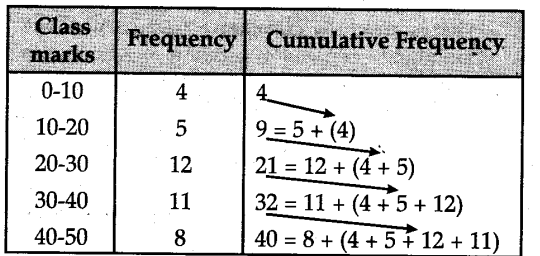# What do you understand by Cumulative Fre-quency Distribution ? Explain with example

The cumulative frequency is calculated by adding each frequency from a frequency distribution table to the sum of its predecessors. The last value will always be equal to the total of all observations, since all frequencies will already have been added to the previous total
The following frequency distribution table gives the marks obtained by 40 students :